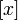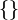# Greatest integer function

The greatest integer function of$x$ is sometimes denoted$[x]$. However, the square brace notation$[]$ is also used in a number of other contexts and should not always be construed as meaning the greatest integer function.
The greatest integer function is related to the fractional part function (sometimes denoted$\{ \}$) as follows: for any$x \in \R$, we have:$\! x = [x] + \{ x \}$